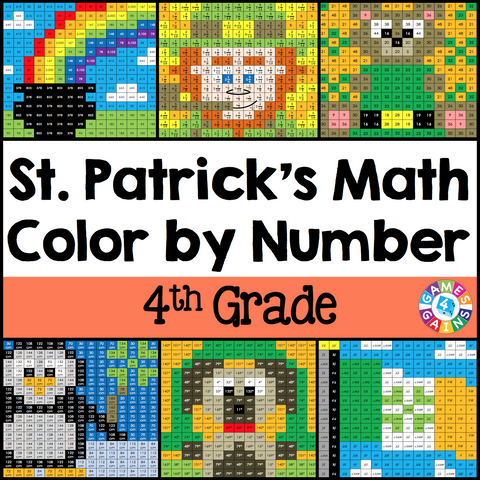## St. Patrick's Day Math Color-by-Number - 4th Grade

• \$480

St. Patrick's Day Math Color-by-Number set comes with 6 St. Patrick's Day color-by-number activities for reviewing 4th grade math skills. This St. Patrick's Day math set is perfect to use in centers, in small groups, or with the whole class!

Included with this St. Patrick's Day Math Color-by-Number set:
Instructions
6 color by number worksheets
6 student problem worksheets
6 teacher answer sheets

Here are the skills covered by these St. Patrick's Day math color-by-number activities:

• Adding and subtracting mixed numbers with like denominators
• Generating a number pattern from a one-step rule
• Converting customary measurements of capacity (larger unit to smaller unit)
• Calculating the perimeter of an irregular figure (including finding missing side dimensions)
• Measuring angles to the nearest degree
• Identifying points, rays, lines, line segments, and types of angles

Want to learn more about what you'll get? Check out the preview to see a sample of the actual pages.